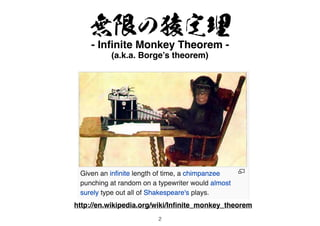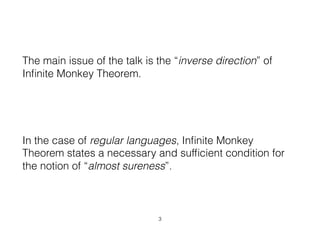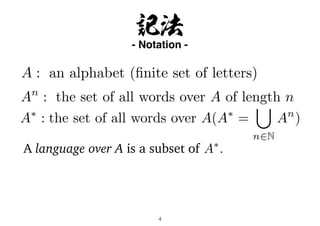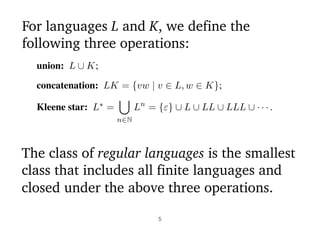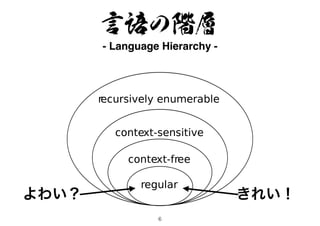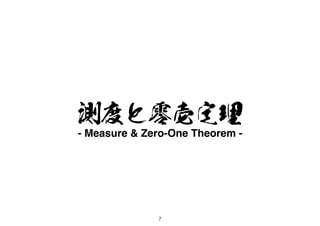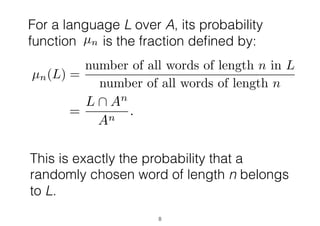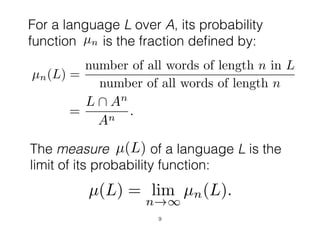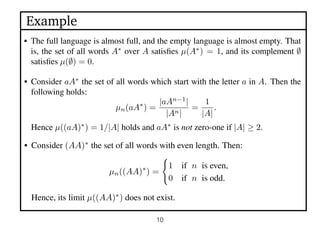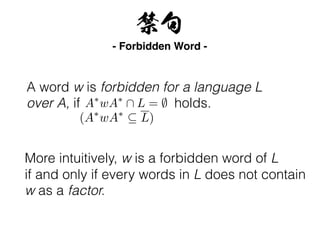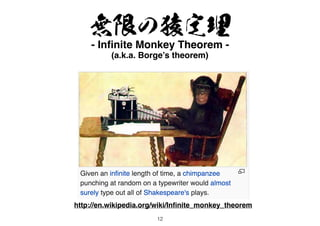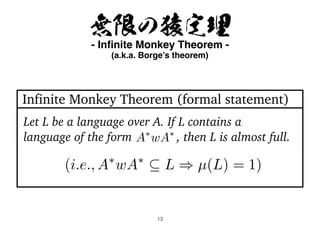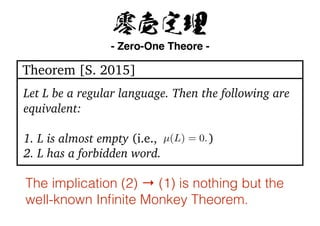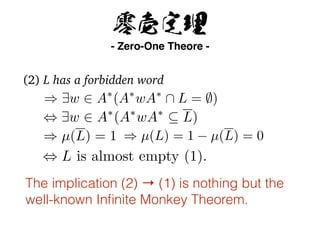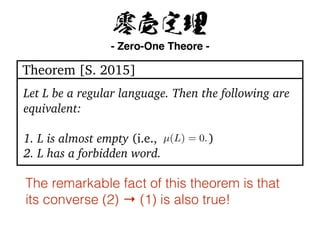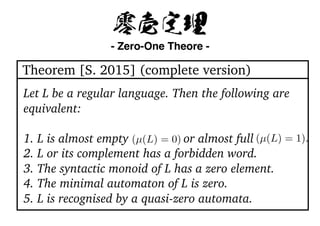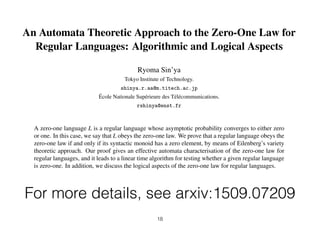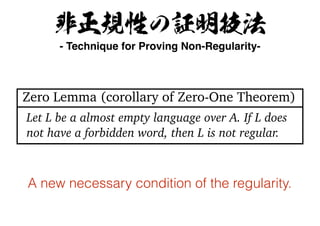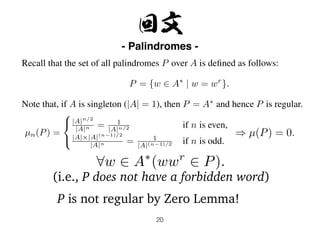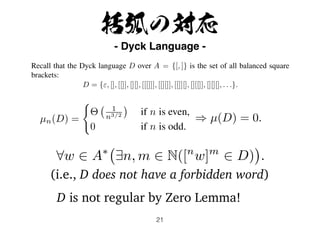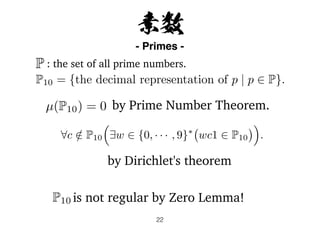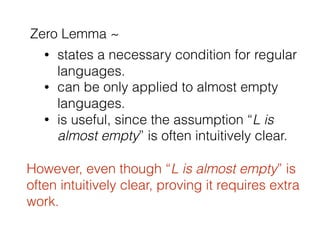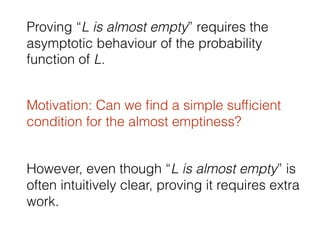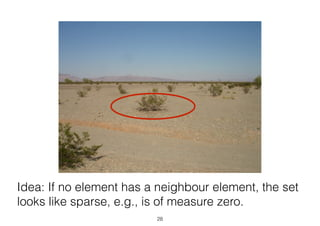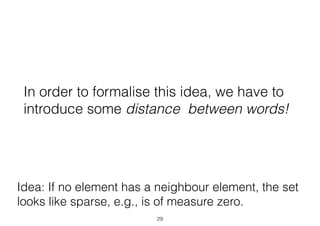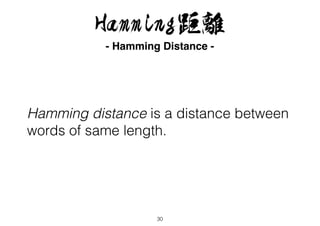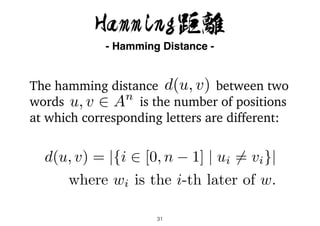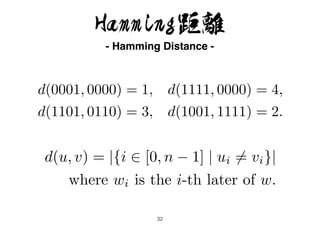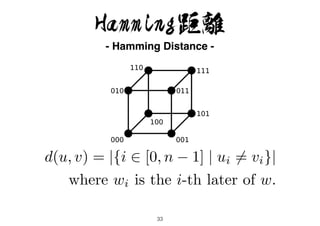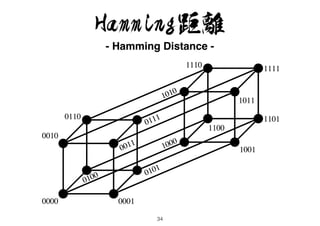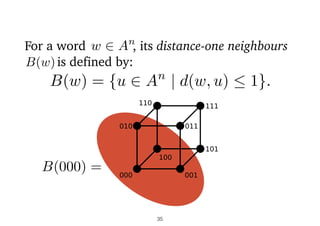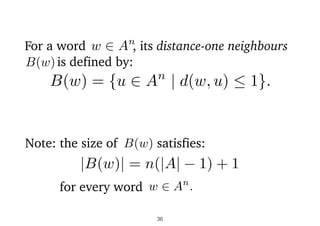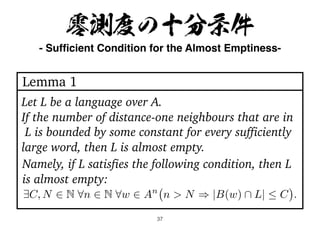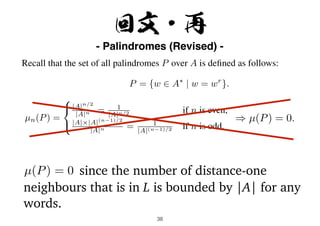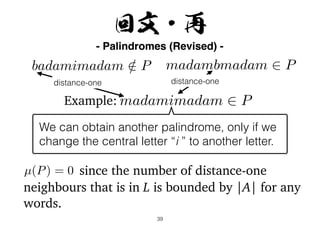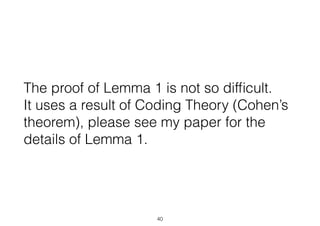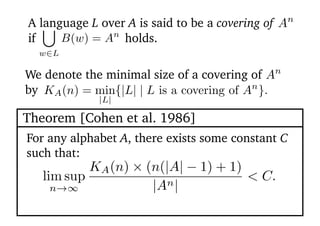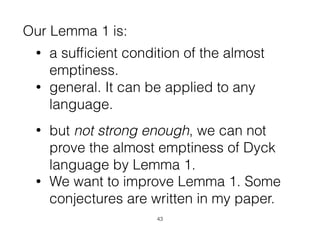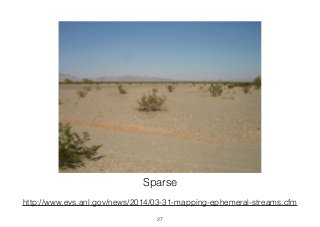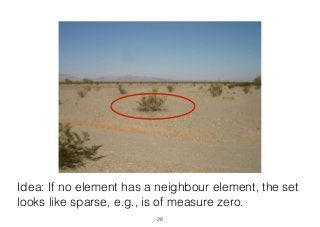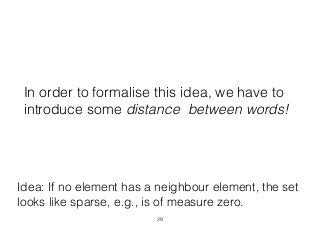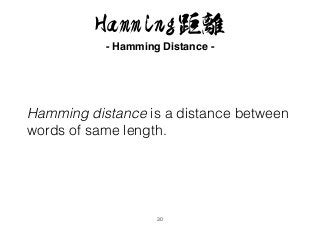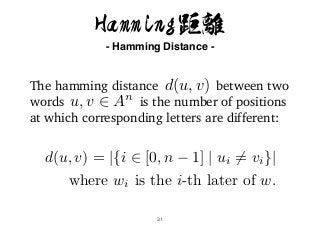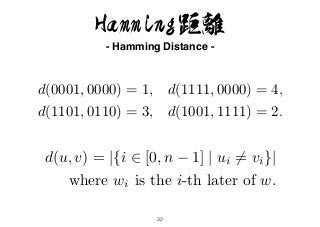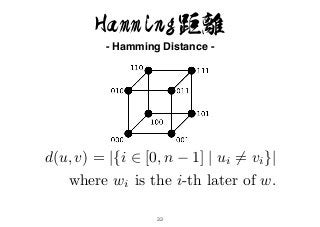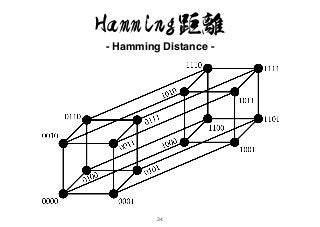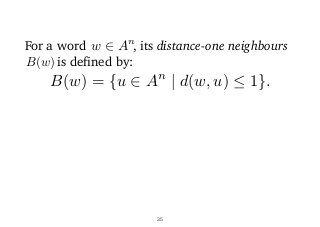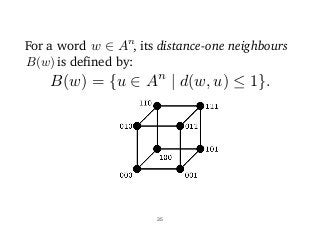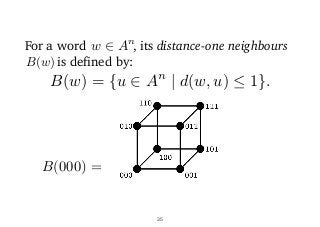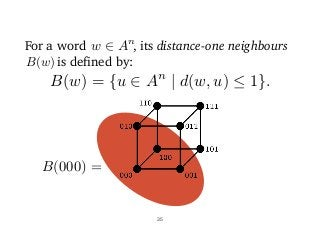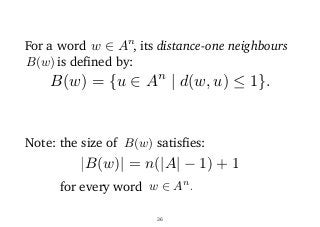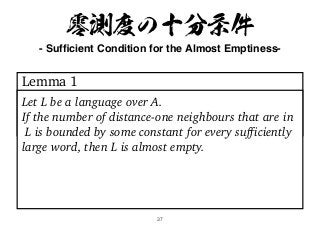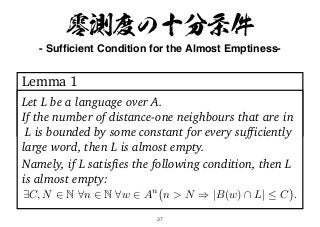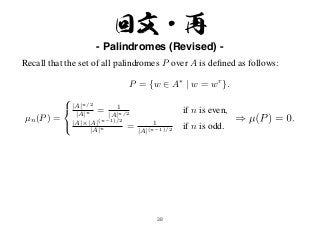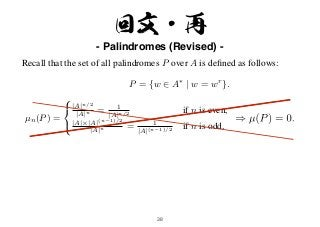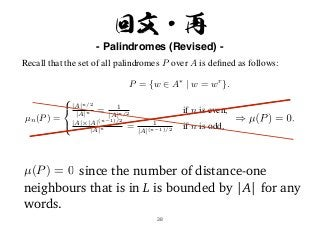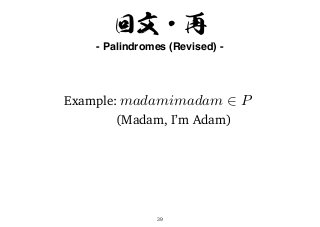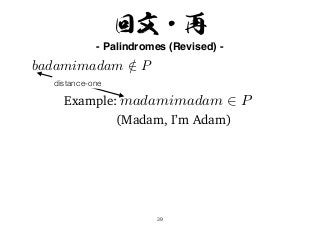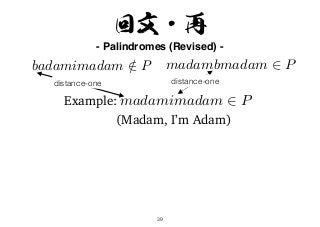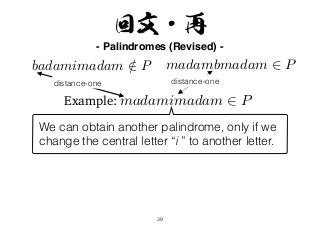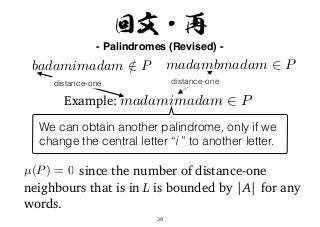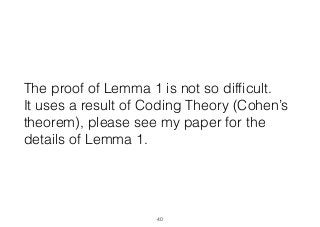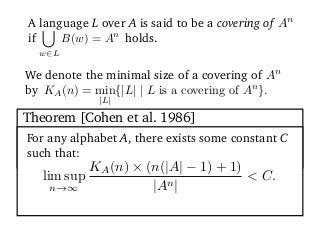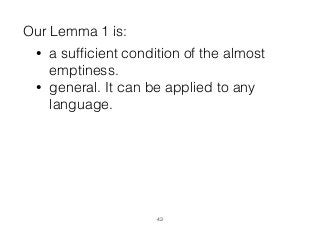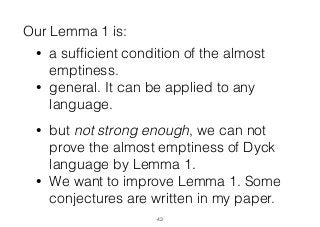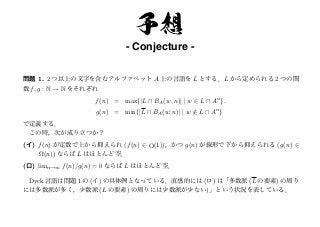1 sur 76

## RecommandéRegular Languagesparmeet834Context free langaugessudhir sharma1.5 & 1.6 regular languages &amp; regular expressionSampath Kumar SdraftManoj KilaruАлексей Чеусов - Расчёсываем своё ЧСВMinsk Linux User Group

### A new technique for proving non regularity based on the measure of a language

1. 言語の測度に基づく非正規性の証明技法 Ryoma Sin’ya Tokyo Institute of Technology, Department of Mathematics and Computer Science. A NEW TECHNIQUE FOR PROVING NON-REGULARITY BASED ON THE MEASURE OF A LANGUAGE
2. 無限の猿定理 - Inﬁnite Monkey Theorem - (a.k.a. Borge’s theorem) http://en.wikipedia.org/wiki/Inﬁnite_monkey_theorem 2
3. The main issue of the talk is the “inverse direction” of Inﬁnite Monkey Theorem. In the case of regular languages, Inﬁnite Monkey Theorem states a necessary and sufﬁcient condition for the notion of “almost sureness”. 3
4. 4 記法 - Notation - A : an alphabet (ﬁnite set of letters) An : the set of all words over A of length n A⇤ : the set of all words over A(A⇤ = [ n2N An ) A language over A is a subset of A⇤ .
5. 5 union: L ∪ K; concatenation: LK = {vw | v ∈ L, w ∈ K}; Kleene star: L∗ = n∈N Ln = {ε} ∪ L ∪ LL ∪ LLL ∪ · · · . For languages L and K, we define the following three operations: The class of regular languages is the smallest class that includes all finite languages and closed under the above three operations.
6. 6 言語の階層 - Language Hierarchy -
7. 6 言語の階層 - Language Hierarchy - よわい？
8. 6 言語の階層 - Language Hierarchy - よわい？ きれい！
9. 測度と零壱定理 - Measure & Zero-One Theorem - 7
10. µn(L) = number of all words of length n in L number of all words of length n For a language L over A, its probability function is the fraction deﬁned by: 8 = |L An | |An| .
11. µn(L) = number of all words of length n in L number of all words of length n This is exactly the probability that a randomly chosen word of length n belongs to L. For a language L over A, its probability function is the fraction deﬁned by: 8 = |L An | |An| .
12. For a language L over A, its probability function is the fraction deﬁned by: µn(L) = number of all words of length n in L number of all words of length n The measure of a language L is the limit of its probability function: µ(L) µ(L) = lim n!1 µn(L). 9 = |L An | |An| .
13. Example 10 The full language is almost full, and the empty language is almost empty. That is, the set of all words A∗ over A satisﬁes µ(A∗ ) = 1, and its complement ∅ satisﬁes µ(∅) = 0.
14. Example 10 Consider aA∗ the set of all words which start with the letter a in A. Then the following holds: µn(aA∗ ) = |aAn−1 | |An| = 1 |A| . Hence µ((aA)∗ ) = 1/|A| holds and aA∗ is not zero-one if |A| ≥ 2. The full language is almost full, and the empty language is almost empty. That is, the set of all words A∗ over A satisﬁes µ(A∗ ) = 1, and its complement ∅ satisﬁes µ(∅) = 0.
15. Example 10 Consider aA∗ the set of all words which start with the letter a in A. Then the following holds: µn(aA∗ ) = |aAn−1 | |An| = 1 |A| . Hence µ((aA)∗ ) = 1/|A| holds and aA∗ is not zero-one if |A| ≥ 2. The full language is almost full, and the empty language is almost empty. That is, the set of all words A∗ over A satisﬁes µ(A∗ ) = 1, and its complement ∅ satisﬁes µ(∅) = 0. Consider (AA)∗ the set of all words with even length. Then: µn((AA)∗ ) = 1 if n is even, 0 if n is odd. Hence, its limit µ((AA)∗ ) does not exist.
16. 禁句 - Forbidden Word - A word w is forbidden for a language L over A, if holds.A⇤ wA⇤ L = ; (A⇤ wA⇤ ✓ L) More intuitively, w is a forbidden word of L if and only if every words in L does not contain w as a factor.
17. 無限の猿定理 - Inﬁnite Monkey Theorem - (a.k.a. Borge’s theorem) http://en.wikipedia.org/wiki/Inﬁnite_monkey_theorem 12
18. 無限の猿定理 - Inﬁnite Monkey Theorem - (a.k.a. Borge’s theorem) 13 Let L be a language over A. If L contains a language of the form , then L is almost full.A⇤ wA⇤ (i.e., A⇤ wA⇤ ✓ L ) µ(L) = 1) Infinite Monkey Theorem (formal statement)
19. 零壱定理 - Zero-One Theore - Let L be a regular language. Then the following are equivalent: 1. L is almost empty (i.e., ) 2. L has a forbidden word. µ(L) = 0. Theorem [S. 2015]
20. 零壱定理 - Zero-One Theore - Let L be a regular language. Then the following are equivalent: 1. L is almost empty (i.e., ) 2. L has a forbidden word. µ(L) = 0. Theorem [S. 2015] The implication (2) → (1) is nothing but the well-known Inﬁnite Monkey Theorem.
21. 零壱定理 - Zero-One Theore - The implication (2) → (1) is nothing but the well-known Inﬁnite Monkey Theorem. (2) L has a forbidden word
22. 零壱定理 - Zero-One Theore - The implication (2) → (1) is nothing but the well-known Inﬁnite Monkey Theorem. (2) L has a forbidden word ) 9w 2 A⇤ (A⇤ wA⇤ L = ;)
23. 零壱定理 - Zero-One Theore - The implication (2) → (1) is nothing but the well-known Inﬁnite Monkey Theorem. (2) L has a forbidden word ) 9w 2 A⇤ (A⇤ wA⇤ L = ;) , 9w 2 A⇤ (A⇤ wA⇤ ✓ L)
24. 零壱定理 - Zero-One Theore - The implication (2) → (1) is nothing but the well-known Inﬁnite Monkey Theorem. (2) L has a forbidden word ) 9w 2 A⇤ (A⇤ wA⇤ L = ;) , 9w 2 A⇤ (A⇤ wA⇤ ✓ L) ) µ(L) = 1
25. 零壱定理 - Zero-One Theore - The implication (2) → (1) is nothing but the well-known Inﬁnite Monkey Theorem. (2) L has a forbidden word ) 9w 2 A⇤ (A⇤ wA⇤ L = ;) , 9w 2 A⇤ (A⇤ wA⇤ ✓ L) ) µ(L) = 1 ) µ(L) = 1 − µ(L) = 0
26. 零壱定理 - Zero-One Theore - The implication (2) → (1) is nothing but the well-known Inﬁnite Monkey Theorem. (2) L has a forbidden word ) 9w 2 A⇤ (A⇤ wA⇤ L = ;) , 9w 2 A⇤ (A⇤ wA⇤ ✓ L) ) µ(L) = 1 ) µ(L) = 1 − µ(L) = 0 , L is almost empty (1).
27. 零壱定理 - Zero-One Theore - Let L be a regular language. Then the following are equivalent: 1. L is almost empty (i.e., ) 2. L has a forbidden word. µ(L) = 0. Theorem [S. 2015] The remarkable fact of this theorem is that its converse (1) → (2) is also true!
28. 零壱定理 - Zero-One Theore - Let L be a regular language. Then the following are equivalent: 1. L is almost empty or almost full 2. L or its complement has a forbidden word. 3. The syntactic monoid of L has a zero element. 4. The minimal automaton of L is zero. 5. L is recognised by a quasi-zero automata. (µ(L) = 0) (µ(L) = 1). Theorem [S. 2015] (complete version)
29. An Automata Theoretic Approach to the Zero-One Law for Regular Languages: Algorithmic and Logical Aspects Ryoma Sin’ya Tokyo Institute of Technology. shinya.r.aa@m.titech.ac.jp ´Ecole Nationale Sup´erieure des T´el´ecommunications. rshinya@enst.fr A zero-one language L is a regular language whose asymptotic probability converges to either zero or one. In this case, we say that L obeys the zero-one law. We prove that a regular language obeys the zero-one law if and only if its syntactic monoid has a zero element, by means of Eilenberg’s variety theoretic approach. Our proof gives an effective automata characterisation of the zero-one law for regular languages, and it leads to a linear time algorithm for testing whether a given regular language is zero-one. In addition, we discuss the logical aspects of the zero-one law for regular languages. For more details, see arxiv:1509.07209 18
30. 非正規性の証明技法 - Technique for Proving Non-Regularity- Let L be a almost empty language over A. If L does not have a forbidden word, then L is not regular. Zero Lemma (corollary of Zero-One Theorem)
31. 非正規性の証明技法 - Technique for Proving Non-Regularity- Let L be a almost empty language over A. If L does not have a forbidden word, then L is not regular. Zero Lemma (corollary of Zero-One Theorem) A new necessary condition of the regularity.
32. 20 回文 - Palindromes - Recall that the set of all palindromes P over A is deﬁned as follows: P = {w ∈ A∗ | w = wr }. Note that, if A is singleton (|A| = 1), then P = A∗ and hence P is regular.
33. 20 回文 - Palindromes - Recall that the set of all palindromes P over A is deﬁned as follows: P = {w ∈ A∗ | w = wr }. Note that, if A is singleton (|A| = 1), then P = A∗ and hence P is regular. µn(P) = ⎧ ⎨ ⎩ |A|n/2 |A|n = 1 |A|n/2 if n is even, |A|×|A|(n−1)/2 |A|n = 1 |A|(n−1)/2 if n is odd.
34. 20 回文 - Palindromes - Recall that the set of all palindromes P over A is deﬁned as follows: P = {w ∈ A∗ | w = wr }. Note that, if A is singleton (|A| = 1), then P = A∗ and hence P is regular. µn(P) = ⎧ ⎨ ⎩ |A|n/2 |A|n = 1 |A|n/2 if n is even, |A|×|A|(n−1)/2 |A|n = 1 |A|(n−1)/2 if n is odd.
35. 20 回文 - Palindromes - Recall that the set of all palindromes P over A is deﬁned as follows: P = {w ∈ A∗ | w = wr }. Note that, if A is singleton (|A| = 1), then P = A∗ and hence P is regular. µn(P) = ⎧ ⎨ ⎩ |A|n/2 |A|n = 1 |A|n/2 if n is even, |A|×|A|(n−1)/2 |A|n = 1 |A|(n−1)/2 if n is odd. 8w 2 A⇤ (wwr 2 P).
36. 20 回文 - Palindromes - Recall that the set of all palindromes P over A is deﬁned as follows: P = {w ∈ A∗ | w = wr }. Note that, if A is singleton (|A| = 1), then P = A∗ and hence P is regular. µn(P) = ⎧ ⎨ ⎩ |A|n/2 |A|n = 1 |A|n/2 if n is even, |A|×|A|(n−1)/2 |A|n = 1 |A|(n−1)/2 if n is odd. 8w 2 A⇤ (wwr 2 P). (i.e., P does not have a forbidden word)
37. 20 P is not regular by Zero Lemma! 回文 - Palindromes - Recall that the set of all palindromes P over A is deﬁned as follows: P = {w ∈ A∗ | w = wr }. Note that, if A is singleton (|A| = 1), then P = A∗ and hence P is regular. µn(P) = ⎧ ⎨ ⎩ |A|n/2 |A|n = 1 |A|n/2 if n is even, |A|×|A|(n−1)/2 |A|n = 1 |A|(n−1)/2 if n is odd. 8w 2 A⇤ (wwr 2 P). (i.e., P does not have a forbidden word)
38. 21 Recall that the Dyck language D over A = {[, ]} is the set of all balanced square brackets: D = {ε, [], [[]], [][], [[[]]], [[][]], [[]][], [][[]], [][][], . . .}. µn(D) = Θ 1 n3/2 if n is even, 0 if n is odd. D is not regular by Zero Lemma! 括弧の対応 - Dyck Language - 8w 2 A⇤ 9n, m 2 N([n w]m 2 D) . (i.e., D does not have a forbidden word)
39. 22 by Prime Number Theorem. is not regular by Zero Lemma! by Dirichlet's theorem : the set of all prime numbers. 素数 - Primes -
40. Zero Lemma ~ • states a necessary condition for regular languages. • can be only applied to almost empty languages. • is useful, since the assumption “L is almost empty” is often intuitively clear.
41. Zero Lemma ~ • states a necessary condition for regular languages. • can be only applied to almost empty languages. • is useful, since the assumption “L is almost empty” is often intuitively clear. However, even though “L is almost empty” is often intuitively clear, proving it requires extra work.
42. Proving “L is almost empty” requires the asymptotic behaviour of the probability function of L. However, even though “L is almost empty” is often intuitively clear, proving it requires extra work.
43. Motivation: Can we ﬁnd a simple sufﬁcient condition for the almost emptiness? Proving “L is almost empty” requires the asymptotic behaviour of the probability function of L. However, even though “L is almost empty” is often intuitively clear, proving it requires extra work.
44. 零測度の十分条件 - Sufﬁcient Condition for the Almost Emptiness- 25
45. http://www.newscientist.com/article/dn10521-forest-growth-is-encouraging-say-researchers/ Dense 26
46. http://www.evs.anl.gov/news/2014/03-31-mapping-ephemeral-streams.cfm Sparse 27
47. Idea: If no element has a neighbour element, the set looks like sparse, e.g., is of measure zero. 28
48. In order to formalise this idea, we have to introduce some distance between words! 29 Idea: If no element has a neighbour element, the set looks like sparse, e.g., is of measure zero.
49. 30 Hamming距離 - Hamming Distance - Hamming distance is a distance between words of same length.
50. 31 Hamming距離 - Hamming Distance - d(u, v) = |{i 2 [0, n − 1] | ui 6= vi}| where wi is the i-th later of w. The hamming distance between two words is the number of positions at which corresponding letters are different: u, v 2 An d(u, v)
51. 32 Hamming距離 - Hamming Distance - d(u, v) = |{i 2 [0, n − 1] | ui 6= vi}| where wi is the i-th later of w. d(0001, 0000) = 1, d(1111, 0000) = 4, d(1101, 0110) = 3, d(1001, 1111) = 2.
52. 33 Hamming距離 - Hamming Distance - d(u, v) = |{i 2 [0, n − 1] | ui 6= vi}| where wi is the i-th later of w.
53. 34 Hamming距離 - Hamming Distance -
54. 35 For a word , its distance-one neighbours is defined by: w 2 An B(w) B(w) = {u 2 An | d(w, u)  1}.
55. 35 For a word , its distance-one neighbours is defined by: w 2 An B(w) B(w) = {u 2 An | d(w, u)  1}.
56. 35 For a word , its distance-one neighbours is defined by: w 2 An B(w) B(000) = B(w) = {u 2 An | d(w, u)  1}.
57. 35 For a word , its distance-one neighbours is defined by: w 2 An B(w) B(000) = B(w) = {u 2 An | d(w, u)  1}.
58. 36 For a word , its distance-one neighbours is defined by: w 2 An B(w) Note: the size of satisfies:B(w) |B(w)| = n(|A| − 1) + 1 for every word w 2 An . B(w) = {u 2 An | d(w, u)  1}.
59. 37 零測度の十分条件 - Sufﬁcient Condition for the Almost Emptiness- Lemma 1 Let L be a language over A. If the number of distance-one neighbours that are in L is bounded by some constant for every sufficiently large word, then L is almost empty.
60. 37 零測度の十分条件 - Sufﬁcient Condition for the Almost Emptiness- Lemma 1 Let L be a language over A. If the number of distance-one neighbours that are in L is bounded by some constant for every sufficiently large word, then L is almost empty. Namely, if L satisfies the following condition, then L is almost empty: 9C, N 2 N 8n 2 N 8w 2 An n > N ) |B(w) L|  C .
61. 38 回文・再 - Palindromes (Revised) - Recall that the set of all palindromes P over A is deﬁned as follows: P = {w ∈ A∗ | w = wr }. Note that, if A is singleton (|A| = 1), then P = A∗ and hence P is regular. µn(P) = ⎧ ⎨ ⎩ |A|n/2 |A|n = 1 |A|n/2 if n is even, |A|×|A|(n−1)/2 |A|n = 1 |A|(n−1)/2 if n is odd.
62. 38 回文・再 - Palindromes (Revised) - Recall that the set of all palindromes P over A is deﬁned as follows: P = {w ∈ A∗ | w = wr }. Note that, if A is singleton (|A| = 1), then P = A∗ and hence P is regular. µn(P) = ⎧ ⎨ ⎩ |A|n/2 |A|n = 1 |A|n/2 if n is even, |A|×|A|(n−1)/2 |A|n = 1 |A|(n−1)/2 if n is odd.
63. 38 回文・再 - Palindromes (Revised) - Recall that the set of all palindromes P over A is deﬁned as follows: P = {w ∈ A∗ | w = wr }. Note that, if A is singleton (|A| = 1), then P = A∗ and hence P is regular. µn(P) = ⎧ ⎨ ⎩ |A|n/2 |A|n = 1 |A|n/2 if n is even, |A|×|A|(n−1)/2 |A|n = 1 |A|(n−1)/2 if n is odd. µ(P) = 0 since the number of distance-one neighbours that is in L is bounded by |A| for any words.